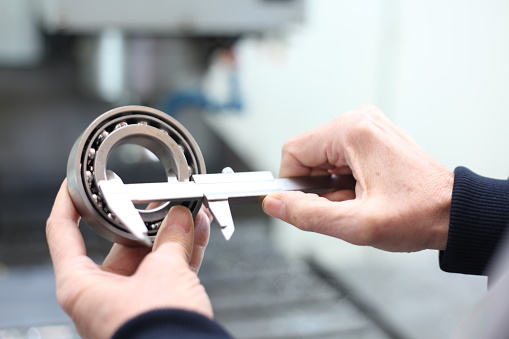## Installing a Bearing

Consider an aluminum bearing with a maximum room-temperature outer diameter of 50.15 mm is to be installed inside of an aluminum mounting plate with a minimum room-temperature bore diameter of 50 mm. If the room temperature is 25°C and the required diametral clearance for assembly is 0.1 mm, what is the minimum temperature the mounting plate must be heated to install a room-temperature bearing? Note, aluminum’s coefficient of linear expansion is 23x10^(-6)/°C.Hint
$$\delta_t=\alpha L(\Delta T)$$$where $$\delta_t$$ is the deformation caused by a change in temperature, $$\alpha$$ is the temperature coefficient of expansion, $$L$$ is the length of the member, and $$\Delta T$$ is the change in temperature. Hint 2 Required diameter change: $$\delta_{diameter}=d_{bearing}-d_{plate}+required\:assy\:clearance$$$
where $$d$$ is the diameter.
Required diameter change:
$$\delta_{diameter}=d_{bearing}-d_{plate}+required\:assy\:clearance$$$where $$d$$ is the diameter. Thus, $$\delta_{diameter}=50.15mm-50mm+0.1mm=0.25\:mm$$$
Required temperature change for the mounting plate’s bore diameter:
$$\delta_t=\alpha L(\Delta T)$$$where $$\delta_t$$ is the deformation caused by a change in temperature, $$\alpha$$ is the temperature coefficient of expansion, $$L$$ is the length of the member, and $$\Delta T$$ is the change in temperature. $$\Delta T=\frac{\delta_{diameter}}{\alpha(L)}=\frac{0.25mm^{\circ}C}{(23\times 10^{-6})(50mm)}=\frac{0.25^{\circ}C}{0.00115}=217.39^{\circ}C$$$
Minimum temperature to which the mounting plate must be heated:
$$T=T_{room}+\Delta T=25^{\circ}C+217.39^{\circ}C=242.39^{\circ}C$$\$
242°C